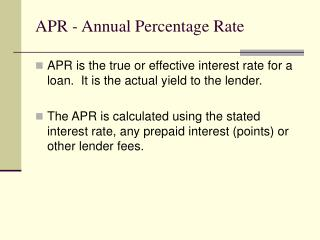DownloadDownload PresentationAPR - Annual Percentage Rate

# APR - Annual Percentage Rate

Télécharger la présentation## APR - Annual Percentage Rate

- - - - - - - - - - - - - - - - - - - - - - - - - - - E N D - - - - - - - - - - - - - - - - - - - - - - - - - - -
##### Presentation Transcript

1. APR - Annual Percentage Rate • APR is the true or effective interest rate for a loan. It is the actual yield to the lender. • The APR is calculated using the stated interest rate, any prepaid interest (points) or other lender fees.

2. Points • Points are loan fees that are viewed as prepaid interest and raise the APR of the loan. One point is 1% of the loan amount.

3. Calculation of APR from a loan with Points • Your are purchasing a residence that has a purchase price of \$250,000. You plan on making a down payment of 20%. Your mortgage lender has agreed to finance the loan at 6% for 30 years, monthly payments, and wants 2 points.

4. Calculate the monthly payment on the loan amount after making the down payment of \$50,000. Calculation of APR from a loan with Points • Loan Amount = \$200,000 • Payment = \$1,199.10 • IR = 6.0 • N = 30 years • P/Y = 12 payments per year

5. Calculation of APR from a loan with Points The amount of the points that is being required is \$200,000 x 0.02 = \$4,000. Therefore the amount of the funded loan is \$200,000 less the \$4,000 = \$196,000.

6. Calculate the APR based on the calculated payment and a funded loan amount of \$196,000. Calculation of APR from a loan with Points • Loan Amount = \$196,000 • PMT = \$1,199.10 • IR = 6.19% APR • N = 30 years • P/Y = 12 payments per year

7. Refinance Analysis The proper perspective for refinancing is to weigh the discounted cash flow savings of the new, lower payment against the cost of the transaction.

8. An Example from the Text Refinance Analysis • Original Loan of \$200,000 at 9% for 30 years with monthly payments • Calculate Monthly Payments • Loan Amount=\$200,000 IR=9.0 N=30 Years, Monthly • PMT= \$1,609.25

9. Refinance Analysis • Refinance the balance after 5 years at 8% with 2 Points and \$1,000 In other loan fees for 25 years with monthly payments. The lender will finance the cost of the points and fees. • What is the payoff amount of the original loan? • Calculating the principal balance following the 60th using the Loan Balance Equation the payment is \$191,760.27. Which is ≈\$191,760

10. Refinance Analysis • AMOUNT OF THE POINTS:\$191,760 x 0.02 = \$3,835 • LOAN FEES = \$1,000 • TOTAL = \$4,835 • AMOUNT OF NEW LOAN = \$191,760 = \$ 4,835TOTAL OF NEW LOAN = \$196,595

11. Refinance Analysis • Calculate the monthly payment for the new loan Loan Amount=\$196,595 IR=8.0 N=25 years Paid monthly • PMT = \$1,517.35 • Since the new loan is paid off at the same time as the original loan, the fact that the new monthly payment is less means the refinance would be profitable.

12. Calculate the Present Value of the Savings from Refinancing Refinance Analysis • Original Payment = \$1,609.25 • New Payment = \$1,517.35 \$ 91.90 • PMT = \$91.90 IR = 8.0 N = 25 Years, Paid monthly • PV = \$11,906.98

13. But what if the new loan is for a term that extends the original term of the loan? Refinance Analysis • If the new loan is for 30 years at 8.0% with 2 points the new loan would extend the payoff date be 5 years. • The monthly payment would be with the Loan Amount=\$ 196,595 IR=8.0 N=30 years with payments occurring monthly • PMT = \$1,442.54

14. Refinance Analysis • The new loan would reduce the payment by \$166.71 per month from the original loan over 25 Years or 300 Payments. • However, there would be an additional 5 years or 60 payments in the amount of \$1,442.54 each.

15. TO EVALUATE THE REFINANCE IN THIS SITUATION, WE NEED TO USE DISCOUNTING. Refinance Analysis • FOR PAYMENTS 1 – 300 (25 YEARS) • Monthly savings=\$166.71 IR=8.0 N=25 years Paid monthly • PV= \$21,599.70 • THIS REPRESENTS THE PRESENT VALUE OF THE SAVINGS OVER THE 25 YEARS

16. Refinance Analysis • NEXT WE NEED TO CALCULATE THE PRESENT VALUE OF THE ADDITIONAL PAYMENTS. • FOR PAYMENTS 301 - 360 (5 YEARS) • PMT= \$1,442.54 IR=8.0 N=5 years, paid monthly • PV= \$71,143.81 • THIS REPRESENTS THE PRESENT VALUE OF THE ADDITIONAL PAYMENTS BACK TO YEAR 25.

17. Refinance Analysis • NEXT WE NEED TO DISCOUNT THIS AMOUNT (\$71,143.81) TO THE PRESENT. • FV= 71,143.81 IR=8.0 N=25 years, paid monthly • PV= \$9,692.38 • THE PRESENT VALUE (BACK TO YEAR 0) OF THE ADDITIONAL PAYMENTS IS \$9,692.38.

18. SO, WHAT IS THE NET RESULT? Refinance Analysis • LET’S EXPRESS THE PV IN TERMS WHERE SAVINGS IS POSITIVE AND AN ADDITIONAL COST IS NEGATIVE. • PV OF SAVINGS FOR 25 YEARS = \$21,599.70 • PV OF ADDITIONAL PAYMENTS FOR 5 YEARS = -\$9,692.38

19. Refinance Analysis • Therefore, the net result is a benefit from refinancing of \$11,907.32 • This means that refinancing would be useful.